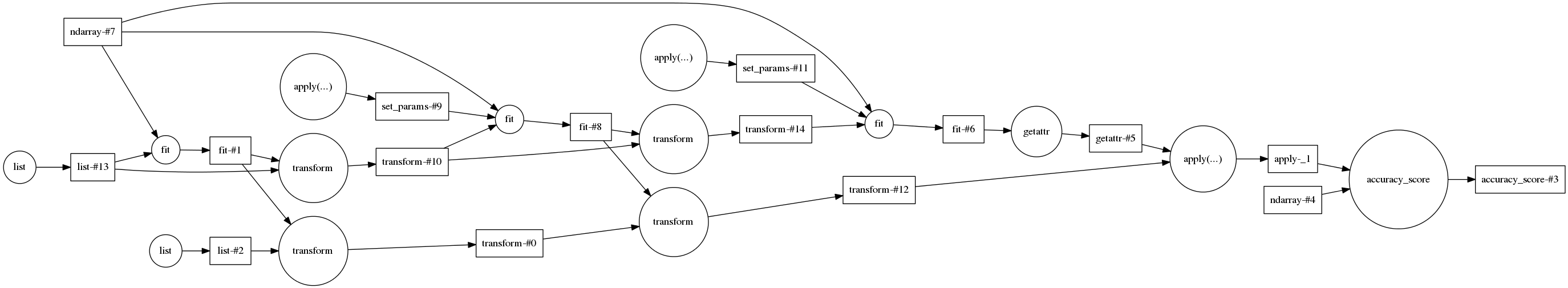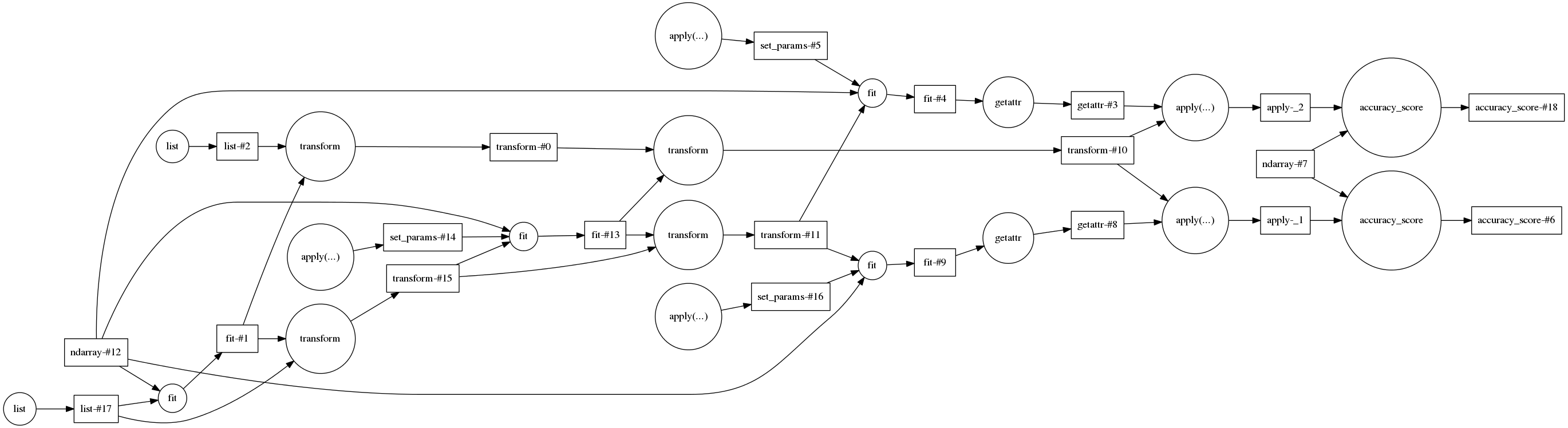## Pipelines and Reuse with dask

##### by Matthew Rocklin

tl;dr: We use dask to accelerate parameter searches over machine learning pipelines by naming consistently.

This work builds on work done with Andreas Mueller, Olivier Grisel, with suggestions by Gael Varoquaux

Disclaimer: this blogpost discusses experimental and buggy code.

## Outline¶

Computational costs of machine learning applications are multiplied by the following:

1. Pipelines of several transformations
2. Parameter searches over each component of those pipelines

Even though the execution of particular components may only take a few seconds, the multiplication of parameter searches over many components may easily blow up full solution time to several minutes or hours.

Fortunately individual computations for a single parameter set can be done in an embarassingly parallel manner. Scikit learn already supports parallel execution in this manner with joblib.

However, if we're clever we can identify and reuse identical computations shared across pipelines with different parameter sets. In many cases this can lead to very striking performance increases that are completely separate from the gains of parallel computing

This post details a trivial copy of sklearn's Pipeline object built with dask and how, by paying careful attention to naming tasks, we're able to drastically speedup parameter search computations, even without parallelism.

## Problem Definition¶

This section poses a scikit-learn problem that we will accelerate in future sections. If you are unfamiliar with scikit-learn you can safely skip details.

#### Consider the following pipeline¶

In :
from sklearn.pipeline import Pipeline
from sklearn.feature_extraction.text import CountVectorizer
from sklearn.feature_selection import SelectFdr
from sklearn.svm import LinearSVC

pipeline = Pipeline([("count", CountVectorizer()),
("select_fdr", SelectFdr()),
("svm", LinearSVC())])


For a set of documents we do the following:

1. Extract features (words) with a count vectorizer
2. Select an important subset of features/words
3. Perform a linear support vector classification

#### Collect newsgroups data¶

We grab some documents from newgroups to play with.

In :
from sklearn.datasets import fetch_20newsgroups

categories = [
'alt.atheism',
'talk.religion.misc',
]

data_train = fetch_20newsgroups(subset='train', categories=categories)
data_test = fetch_20newsgroups(subset='test', categories=categories)


#### Train/test split:¶

In :
X_train, y_train = data_train.data, data_train.target
X_test, y_test = data_test.data, data_test.target


#### Fit, predict, score¶

We finally fit our pipeline on the training data, predict on the testing data, and then score how well our pipeline performs.

In :
%%time
pipeline.fit(X_train, y_train).score(X_test, y_test)

CPU times: user 581 ms, sys: 0 ns, total: 581 ms
Wall time: 581 ms

Out:
0.77017543859649118

77% classification accuracy after less than a second of computation time. Great.

#### Parameter Searches¶

We can achieve better scores by tuning the parameters of each of the components of the pipeline. The default parameters provided by scikit-learn are quite sane but datasets vary and parameter tuning helps.

So we're going to choose a few parameters in the second and third parts of our pipeline and consider all possible combinations.

Disclaimer: to make this problem more explicit we avoid sklearn's GridSearch object and just use a for loop. Please don't do this in practice.

In :
import numpy as np
parameters = [{'select_fdr__alpha': alpha, 'svm__C': C}
for alpha in [0.05, 0.01, 0.1, 0.2]
for C in np.logspace(-3, 2, 6)]

In :
%%time
scores = [pipeline.set_params(**params)
.fit(X_train, y_train)
.score(X_test, y_test)
for params in parameters]

CPU times: user 11.9 s, sys: 0 ns, total: 11.9 s
Wall time: 11.9 s

In :
{'best-score': max(scores),
'best-parameters': parameters[np.argmax(scores)]}

Out:
{'best-parameters': {'select_fdr__alpha': 0.2, 'svm__C': 0.01},
'best-score': 0.79649122807017547}

So modest parameter searching yield a bit of an improvement at a 20x cost increase. For problems of real size this change can grow significantly larger.

We've built a tiny dask Pipeline object to mimic sklearn's.

It looks the same but has a couple of important differences

1. It does not support mutation, you must reassign after .fit and .set_params calls. E.g.

  sklearn:            pipeline.fit(X, y)



In this post we only use method chaining, so this won't come up.

2. Results are lazy dask objects, we'll need to do a final compute step get the full results.
In :
# from sklearn.pipeline import Pipeline

pipeline = Pipeline([("count", CountVectorizer()),
("select_fdr", SelectFdr()),
("svm", LinearSVC())])


When used for a single parameter set it's a silly use of dask. The computation isn't very parallelizable.

In :
from dask.base import visualize
params1 = parameters
score1 = (pipeline.set_params(**params1)
.fit(X_train, y_train)
.score(X_test, y_test))

visualize(score1, rankdir='LR')

Out:However, when we combine two of these objects something wonderful happens, almost nothing changes.

In :
params2 = parameters
score2 = (pipeline.set_params(**params2)
.fit(X_train, y_train)
.score(X_test, y_test))

visualize(score1, score2, rankdir='LR')

Out:Almost nothing changes because our parameter sets are actually pretty similar. There are no parameters in the first component of the pipeline, CountVectorizer, and the second component, SelectFDR, has the same value for both parameter sets. We only need to recompute the last component, the LinearSVC.

We accelerate our computation by identifying and avoiding redundant computations that are shared across multiple runs.

In :
parameters, parameters

Out:
({'select_fdr__alpha': 0.05, 'svm__C': 0.01},
{'select_fdr__alpha': 0.05, 'svm__C': 0.10000000000000001})

## Full Computation¶

OK, we've talked enough, lets run the entire computation.

In :
from dask.imperative import compute
from dask.async import get_sync  # no parallelism

In :
%%time
scores = [pipeline.set_params(**params)
.fit(X_train, y_train)
.score(X_test, y_test)
for params in parameters]

result = compute(scores, get=get_sync)

CPU times: user 1.87 s, sys: 16 ms, total: 1.88 s
Wall time: 1.88 s

In :
{'best-score': max(result),
'best-parameters': parameters[np.argmax(result)]}

Out:
{'best-parameters': {'select_fdr__alpha': 0.2, 'svm__C': 0.01},
'best-score': 0.79649122807017547}

A modest 3x increase here rather than 20x.

A large part of this increase is still in dask hashing overhead, which we can remove by pre-specifying our arrays as dask values.

In :
%%time
X_train, y_train, X_test, y_test = map(value, [X_train, y_train, X_test, y_test])

scores = [pipeline.set_params(**params)
.fit(X_train, y_train)
.score(X_test, y_test)
for params in parameters]

result = compute(scores, get=get_sync)

CPU times: user 1.24 s, sys: 0 ns, total: 1.24 s
Wall time: 1.24 s

In :
visualize(*scores, rankdir='LR')

Out: